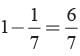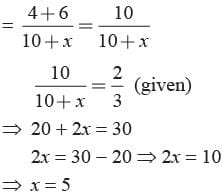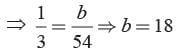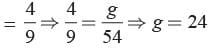# Math Olympiad Test: Probability- 2 - Class 10

Test Description

## 15 Questions MCQ Test Olympiad Preparation for Class 10 - Math Olympiad Test: Probability- 2

Math Olympiad Test: Probability- 2 for Class 10 2023 is part of Olympiad Preparation for Class 10 preparation. The Math Olympiad Test: Probability- 2 questions and answers have been prepared according to the Class 10 exam syllabus.The Math Olympiad Test: Probability- 2 MCQs are made for Class 10 2023 Exam. Find important definitions, questions, notes, meanings, examples, exercises, MCQs and online tests for Math Olympiad Test: Probability- 2 below.
 1 Crore+ students have signed up on EduRev. Have you?
Math Olympiad Test: Probability- 2 - Question 1

### The probability that it will rain today is 0.76. What is the probability that it will not rain today?

Detailed Solution for Math Olympiad Test: Probability- 2 - Question 1

Let E be the event that it will rain today.
P(E) = 0.76
P (That it will not rain today) = 1 - 0.76
= 0.24

Math Olympiad Test: Probability- 2 - Question 2

### A bag contains 19 balls having numbers 1, 2, 3, . . . . . . . 19 respectively. A ball is drawn at random from the bag, what is the probability that the number on the ball is a prime number?

Detailed Solution for Math Olympiad Test: Probability- 2 - Question 2

Total number of possible outcomes = 19 Out of the given numbers, prime numbers are –2, 3, 5, 7, 11, 13, 17, 19
P (the number on the ball is a prime) = 8/19

Math Olympiad Test: Probability- 2 - Question 3

### There are 35 tickets numbered 1, 2, 3, . . . 35. One ticket is drawn at random. What is the probability that the number on the ticket is a multiple of 3 or 5.

Detailed Solution for Math Olympiad Test: Probability- 2 - Question 3

1, 2, 3, . . . . 35
The multiples of 3 or 5 = 3, 5, 6, 9, 10, 12, 15, 18, 20, 21, 24, 25, 27, 30, 33, 35
P (ticket is a multiple of 3 or 5) = 16/35

Math Olympiad Test: Probability- 2 - Question 4

Two dice are thrown simultaneously. What is the probability of getting 9 as the sum of two numbers that turn up?

Detailed Solution for Math Olympiad Test: Probability- 2 - Question 4

Total number of possible outcomes = 36 E = The sum of two numbers be 9 is as (3, 6), (6, 3), (4, 5), (5, 4)
P(E) = 4/36 = 1/9

Math Olympiad Test: Probability- 2 - Question 5

A man calculates that the probability of his winning the first prize in a lottery is 0.08. If 6000 tickets are sold, how many tickets has he bought?

Detailed Solution for Math Olympiad Test: Probability- 2 - Question 5

Given, total number of tickets = 6000
Probability of her winning = 0.08
Total number of tickets she bought = 6000 × 0.08 = 480

Math Olympiad Test: Probability- 2 - Question 6

A die is thrown 350 times and the score of 6 obtained 28 times. Find the probability of getting a score under 6.

Detailed Solution for Math Olympiad Test: Probability- 2 - Question 6

Total number of outcomes = 350
No. of score of 6 obtained = 28
No. of score under 6 obtained = 350 - 28 = 322
Probability of getting the score of under 6 = 322/350 = 23/25

Math Olympiad Test: Probability- 2 - Question 7

What is the probability that a number selected at random from the numbers 1, 2, . . . . . 35 is not a multiple of 7?

Detailed Solution for Math Olympiad Test: Probability- 2 - Question 7

Numbers are 1, 2, 3, .......... 35
Total no. of possible outcomes = 35
E = The number which is multiple of 7
= 7, 14, 21, 28, 35
P(E) = 5/35 = 1/7
P (Not multiple of 7) =Math Olympiad Test: Probability- 2 - Question 8

In a class, there are 35 boys and 15 girls. What is the probability of a randomly selected student of the class to be a girl?

Detailed Solution for Math Olympiad Test: Probability- 2 - Question 8

Total no. of students = 35 + 15 = 50
Probability that selected student is a girl = 15/50 = 3/10

Math Olympiad Test: Probability- 2 - Question 9

A box contains 6 green balls, 4 red balls and some white balls. If the probability of not drawing a white ball in one draw is 2/3, what is the number of white balls?

Detailed Solution for Math Olympiad Test: Probability- 2 - Question 9

Let no. of white balls = x
Total no. of balls = 6 + 4 + x = 10 + x
Probability of drawing not a white ballMath Olympiad Test: Probability- 2 - Question 10

In a pack of 52 playing cards, the king, the queen, the Jack and 10 are lost, All these cards are of spade. A card is drawn from the remaining well shuffled back. Find the probability of getting a king.

Detailed Solution for Math Olympiad Test: Probability- 2 - Question 10

Remaining cards = 52 - 4 = 48
There are 3 kings
∴ P (getting a king) = 3/48 = 1/16

Math Olympiad Test: Probability- 2 - Question 11

A Jar contains 54 marbles each of which is blue, green or white. The probability of getting a blue marble at random from the Jar is 1/3 and the probability of getting a green marble at random is 4/9. What is the number of white marbles?

Detailed Solution for Math Olympiad Test: Probability- 2 - Question 11

Let there are b blue, g green and w white marbles.
b + g + w = 54
P (getting a blue marble) = b/54P (getting a green marble)No. of while marbles = 54 - (18 + 24) = 54 - 42 = 12

Math Olympiad Test: Probability- 2 - Question 12

In a bag, there are 70, Rs.1 coin, 30, Rs. 2 coin and 50, Rs. 5 coin. One coin is drawn at random. What is the probability that it will not be a Rs. 5 coin?

Detailed Solution for Math Olympiad Test: Probability- 2 - Question 12

Total no. of possible outcomes
= 70 + 30 + 50
= 150
No. of Rs. 5 coin = 50
No. of coins of Rs. 1 & Rs. 2 coin = 70 + 30 = 100
P (will not be Rs. 5 coin) = 100/150 = 2/3

Math Olympiad Test: Probability- 2 - Question 13

A letter is chosen at random from the letter of word communication. What is the probability that the chosen letter is a vowel?

Detailed Solution for Math Olympiad Test: Probability- 2 - Question 13

Total no. of letters = 13
No. of vowel letters = 6
Required probability = 6/13

Math Olympiad Test: Probability- 2 - Question 14

A letter is chosen at random from the letter of word concentration. What is the probability that the chosen letter is a consonant?

Detailed Solution for Math Olympiad Test: Probability- 2 - Question 14

Total no. of letters = 13
No. of consonant letters = 8
Required probability = 8/13

Math Olympiad Test: Probability- 2 - Question 15

500 tickets of a lottery were sold. There are 15 prizes on these tickets. If Mohan has purchased one ticket, what is the probability that he will win a price?

Detailed Solution for Math Olympiad Test: Probability- 2 - Question 15

Total no. of tickets = 500
Total no. of prizes = 15
P (win a prize) = 15/500 = 3/100 = 0.03

## Olympiad Preparation for Class 10

11 videos|36 docs|201 tests